# UNITS MASTERInnovative Unit Conversion Calculator

Work smarter and get instant results

Need fast, easy and accurate unit calculator and unit converter?
UNITS MASTER is a smart and innovative solution for unit calculation and conversion that saves your time and helps you avoid calculation mistakes. Due to the built-in intelligent data analyzing algorithm you may not only perform complex calculations but also easily adapt the app to your needs.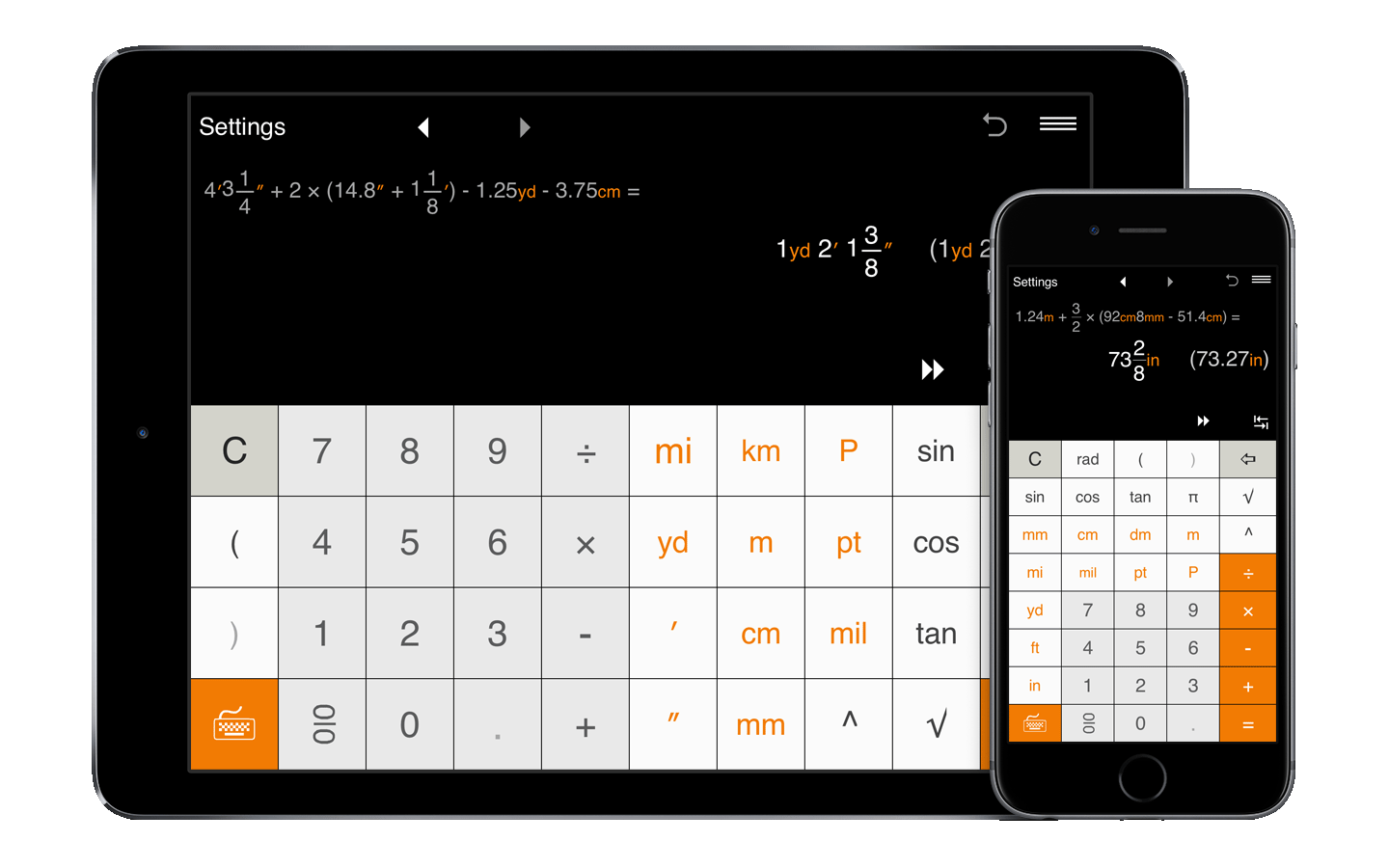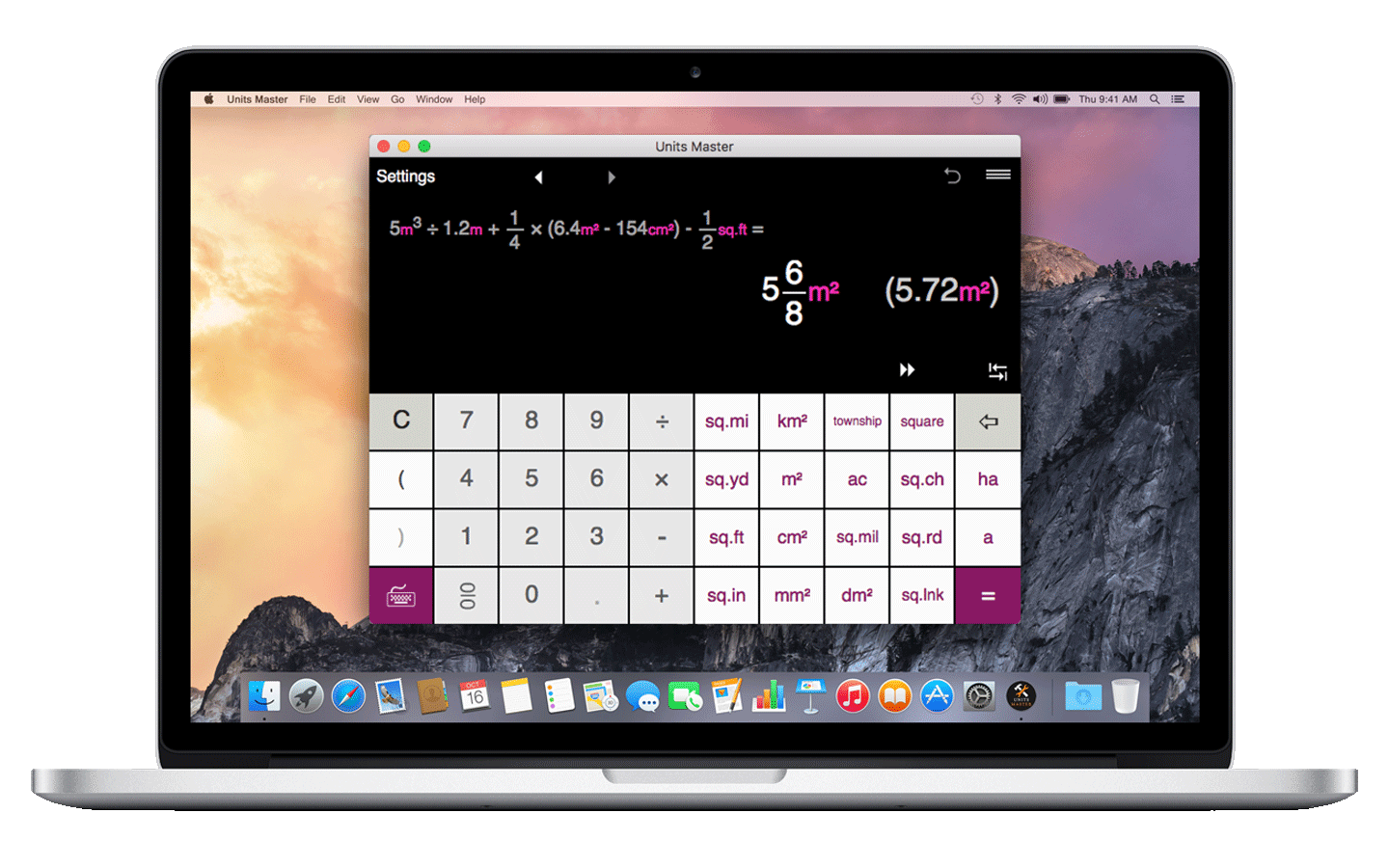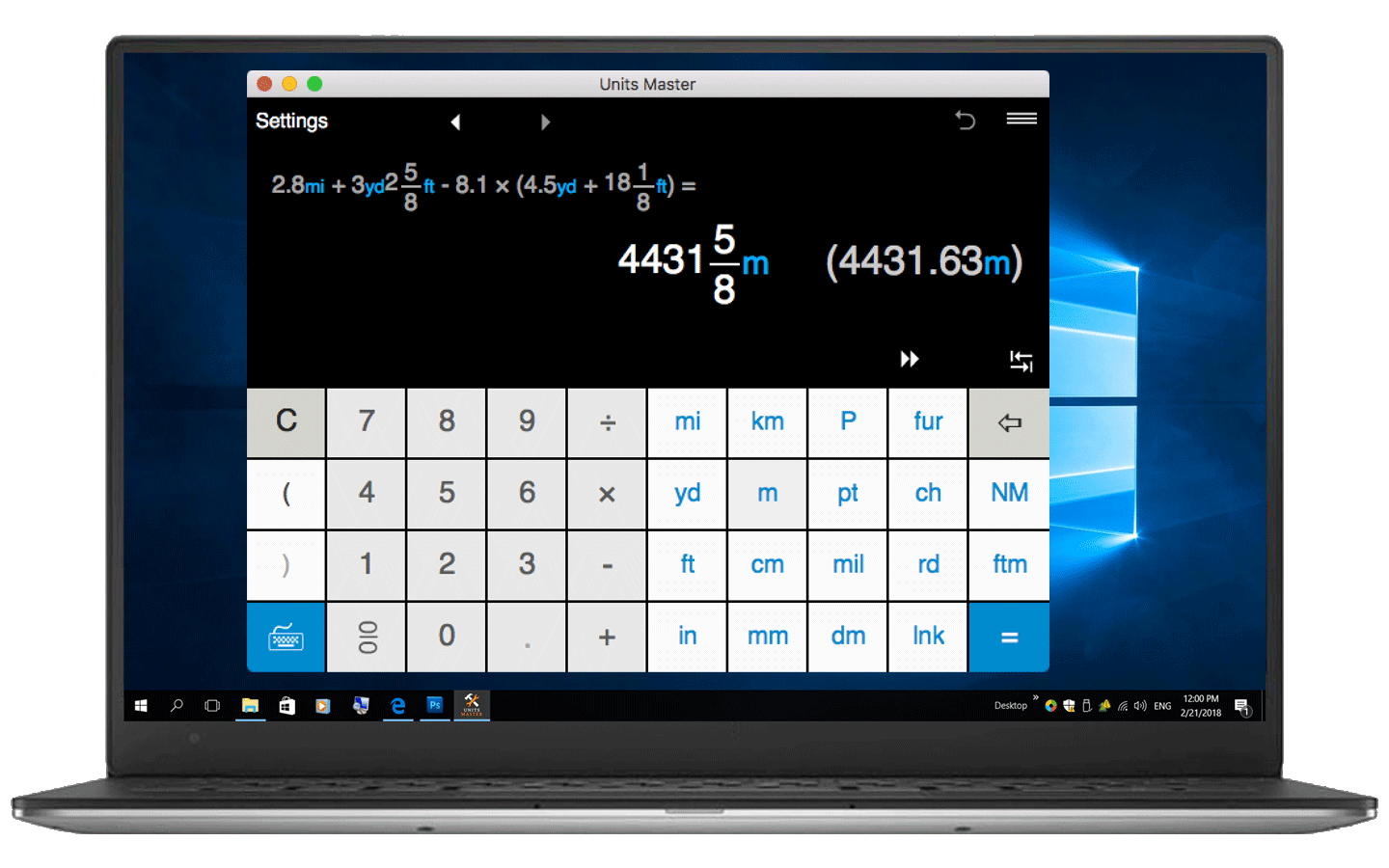Perfect for engineering, construction, building, scientific, technical and mathematical calculations with units, dimensional math and analysis or just unit conversion.

## Solve unit calculations and conversions on your smartphone, tablet or computer

### Full-featured unit calculator and converter

The Units Master App is a full-featured calculator and converter with clean interface and powerful solutions for calculating in fractional a decimal units in both US/imperial and metric systems.

### Quick to adjust and easy to use

The app provides simple input for units and fraction/decimal math, supports a natural display for fractions and shows both the expression and the result so you can easily check the expression and quickly identify and fix any input errors.

### Everyday and advanced unit calculations

Ideal for engineers, contractors, builders, architects, carpenters, designers, scientists, students and anyone who needs to perform unit conversion and calculation on a daily basis.

### Tailor the app to your needs

With Units Master, you may easily get the answer in desired unit(s) in fractional and decimal forms, round results to usable fractions, choose the default output format and more.

## Units calculation

• Instantly calculate using 36 built-in standard (US) and metric length, distance and area units.
• Intuitive interface for units input.
• Calculate in fractions and decimals.
• Calculate linear and square measurements in metric units.
• Calculate linear and square measurements in US/imperial units, including feet-inch-fractions, inch fractions, decimal feet, decimal inches and more.
• Perform calculations when metric and US units are mixed.
• Convert results between different units with one click.
• Get the answer in desired unit(s) in a fractional and decimal form.
• The app displays both the expression and the result at the same time.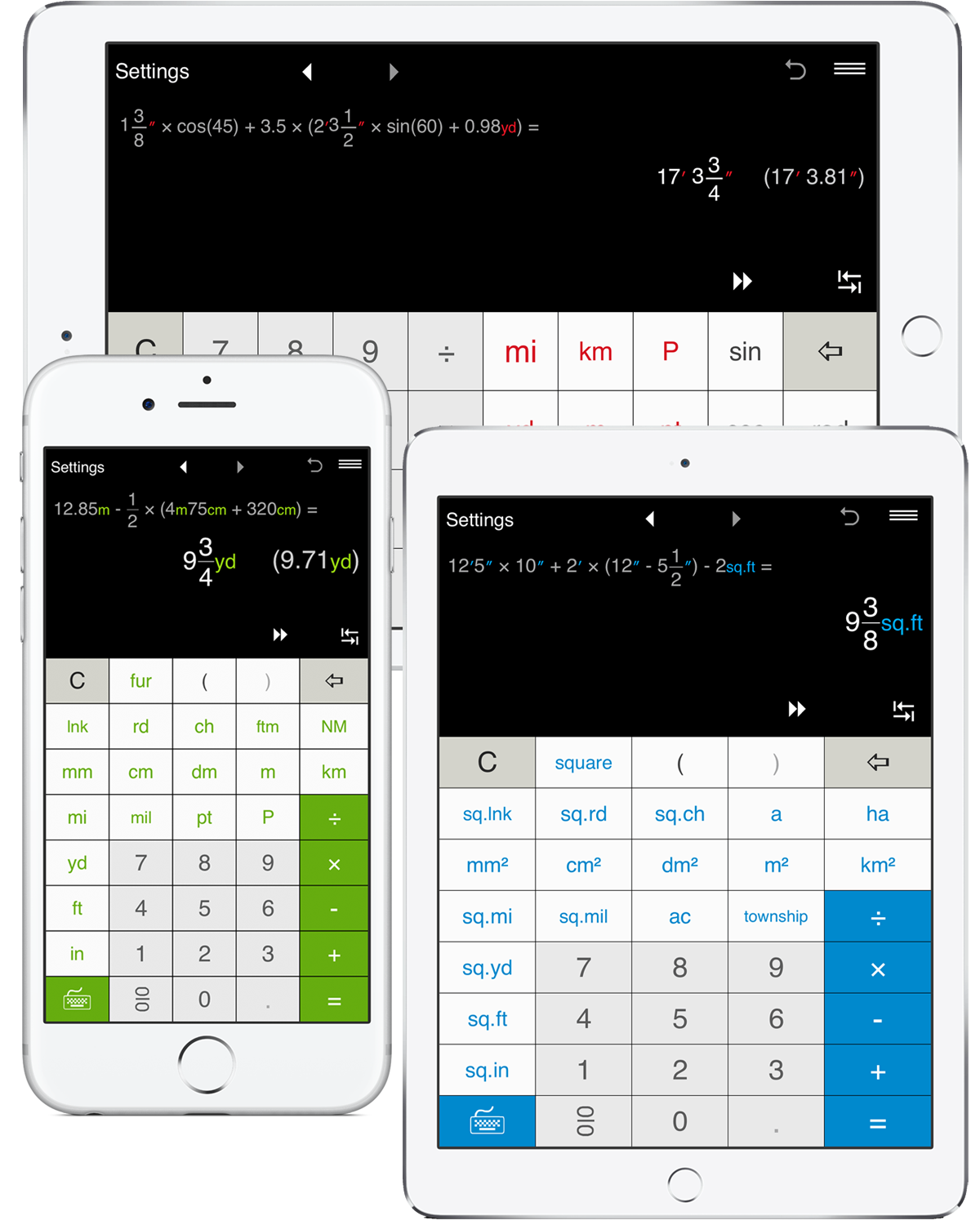## Professional unit conversion calculator

• More than 1000 conversion combinations.
• Convert between fractions and decimals:
- decimal to fraction conversion
- fraction to decimal conversion
• Convert between US, Imperial and metric length and area units, including commonly used inches/centimeters/millimeters, feet/yards/meters, square inches/square feet/m² and other length and area units conversions.
• Convert between units in the same system:
- conversion between metric units
- conversion between US units
• Fractional and decimal unit conversion, including foot-inch-fraction to decimal format conversion.
• One-click result conversion by clicking the unit button you want the result value converted to.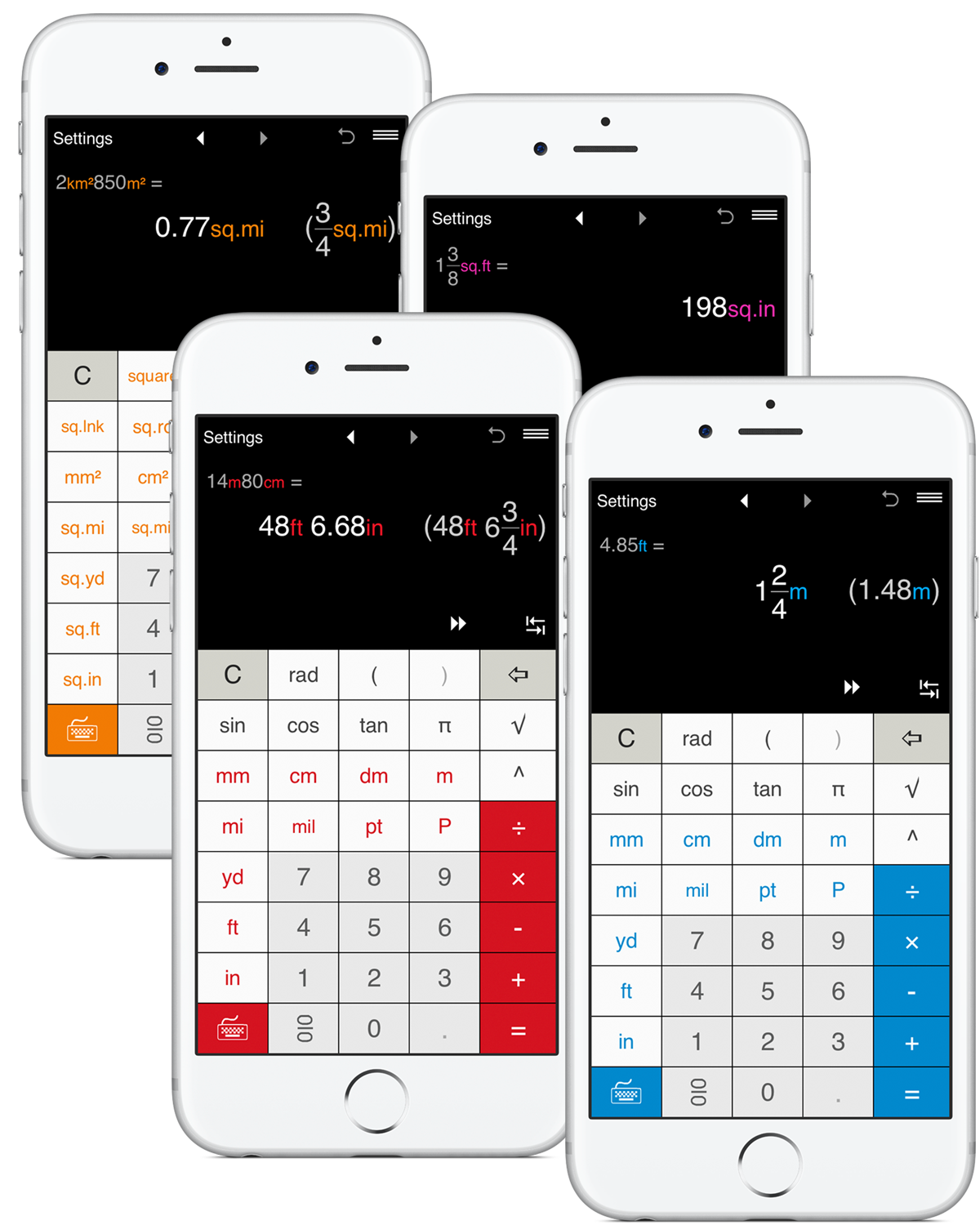## Works as a standard calculator

• Addition, subtraction, multiplication and division.
• Calculations with parentheses, including nested parentheses.
• Fraction and decimal math.
• Powers, trigonometric functions.
• Intuitive fraction input.
• Constants: π (pi).
• Trigonometric calculations in Degrees and Radians.
• Square Root.
• Fraction to decimal and decimal to fraction conversion.
• Reduce fractions and mixed numbers.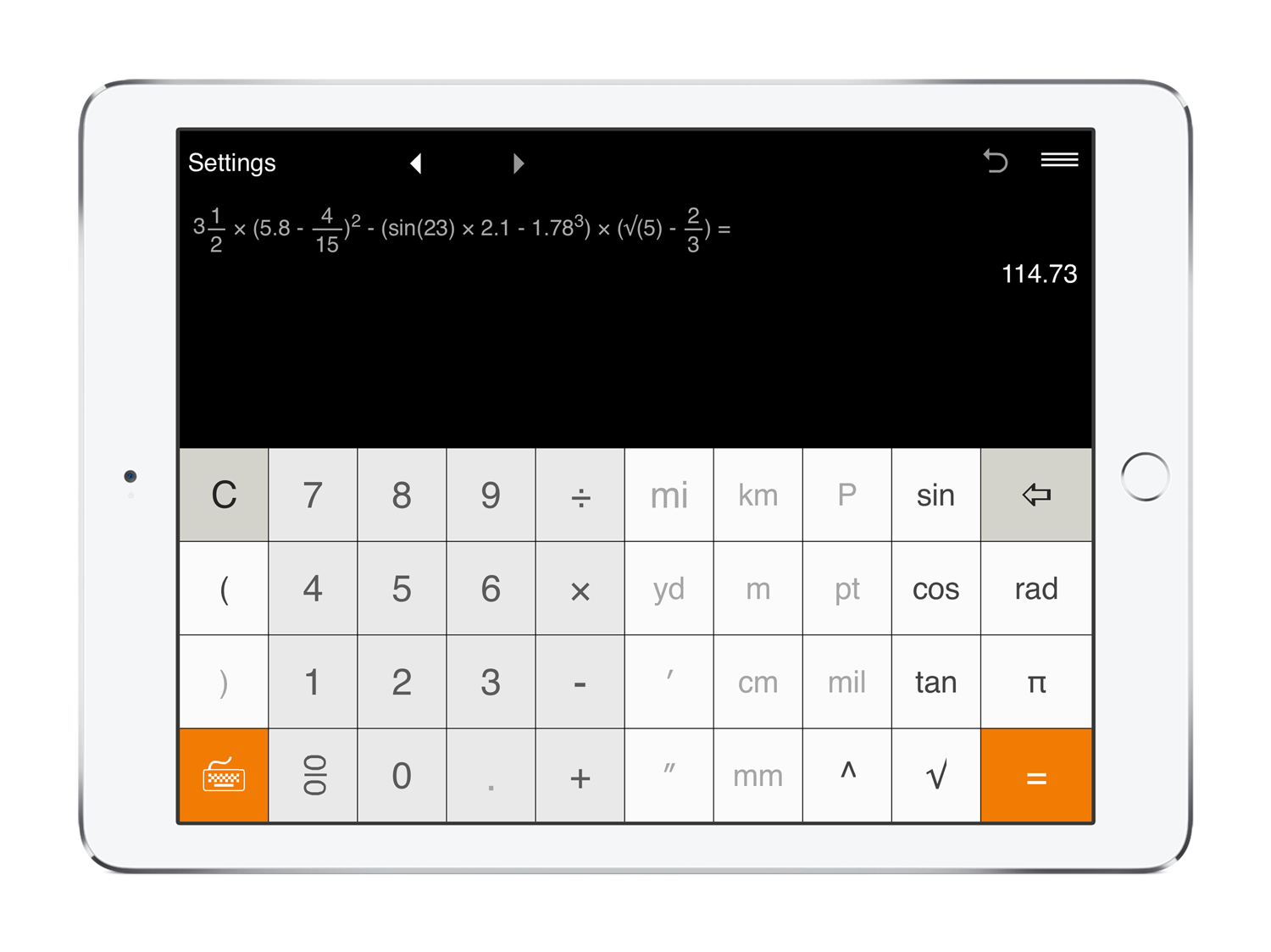## Settings

• Three output modes for results: Automatic, US (imperial) and Metric.
• Round results:
- Decimal numbers can be rounded to 0-8 of significant figures. You can set up the app to round decimals to tenths, hundredths, etc. By default, the result is rounded to 10ths.
- For fractions, you may round the results to the nearest 1/2, 1/4, 1/8, 1/12, 1/16, 1/32, 1/64, 1/128, 1/256. If you do not need to round fractions, just select "-".
• 7 color themes.
• Use the app in either portrait or landscape mode.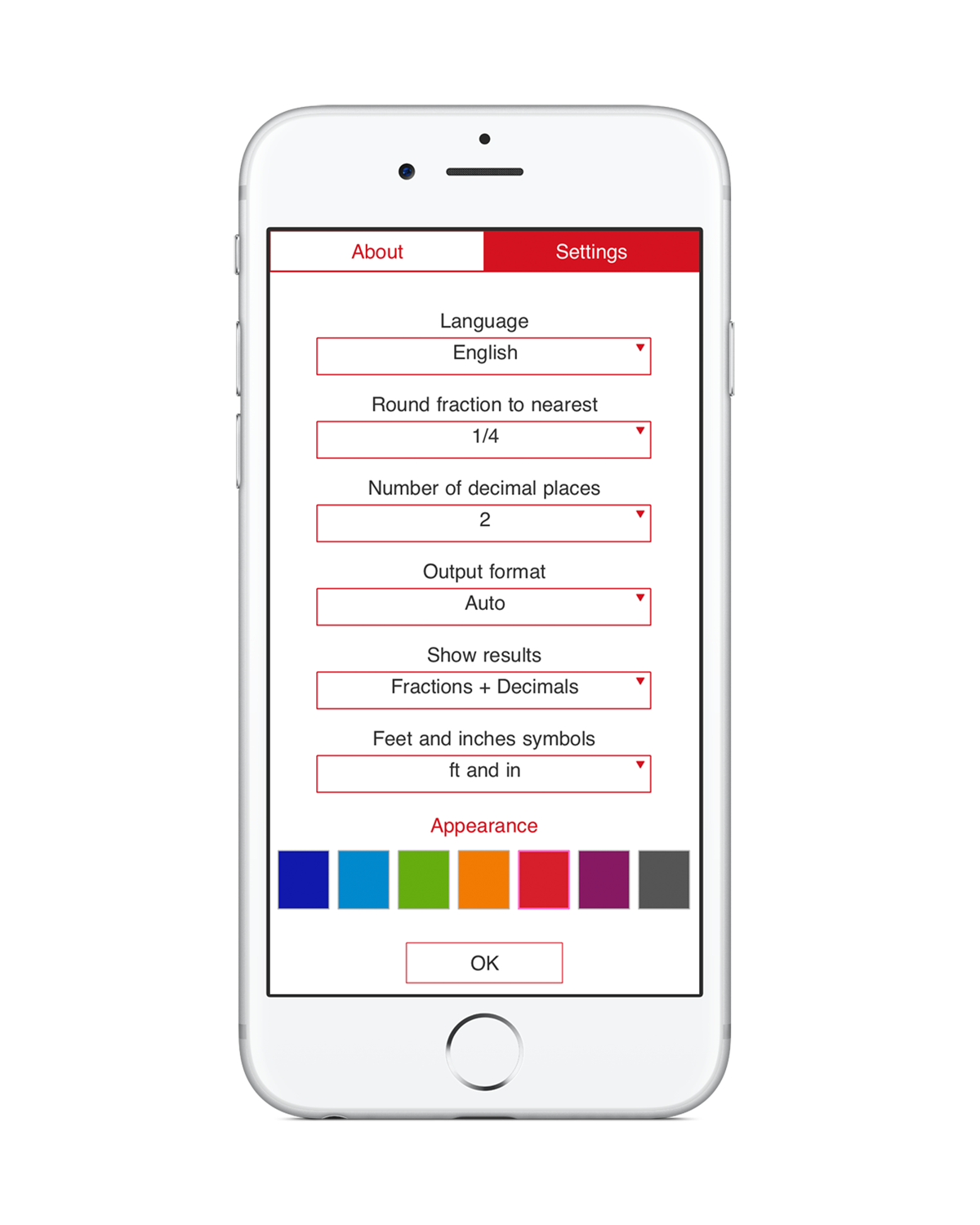### Special Functions• Intuitive interface for fraction input.• Switch between the keyboards.• Convert results between standard and metric systems.• Convert results between units within the same system.• Back and Forward buttons to find and recall recent calculations.• 'Undo' for the Clear command.• History Tape to view and share calculations.• Share calculation results via email.

CS - Čeština

DE - Deutsch

DK - Dansk

EL - Ελληνικά

EN - English

ES - Español

FI - Suomi

FR - Français

IT - Italiano
JP - 日本語

KR - 한국어

NL - Nederlands

NO - Norsk (bokmål)

PT - Português

RU - Русский

SV - Svenska

TR - Türkçe

ZH - 中文